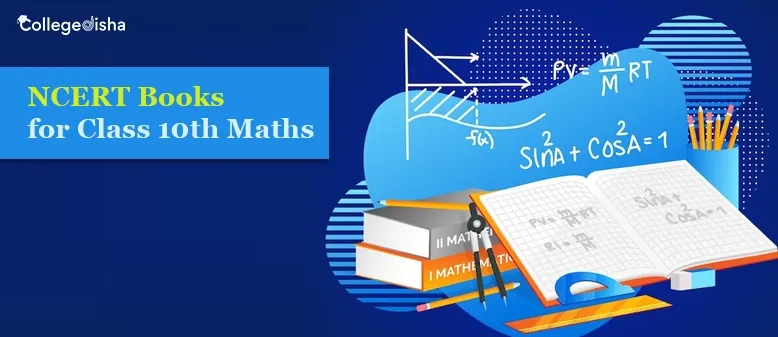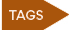# NCERT Books for Class 10th Maths - Check NCERT Class 10th Maths Syllabus

Update on 01 Mar, 23## NCERT Books for 10th Maths:

Mathematics is one of the few subjects that a student studies and learns throughout his or her schooling life. They start with math from their first grade and continue studying it through to 12th in some cases. Class 10th Maths is one of the basic foundational classes that improves and makes the sound base of a student in the subject.

The students who wish to opt for math in their next class, that is, class 11th or 12th, must pay close attention in this class and build a strong foundational base of mathematics so that they can easily grasp the deeper level mathematics of the higher classes. It is a subject that needs a lot of practice, memorization, and a certain amount of dedication to be able to clear doubts and understand concepts.

Students can easily score marks if they study mathematics every day in an orderly manner. There are many NCERT Books for 10th Maths and students can also avail themselves of the help of reference books for studying. The books cover all the topics from Number system, Algebra, Coordinate Geometry, etc. It comprehensively details all the foundational knowledge students must gain to understand the concepts clearly. With exercises and practice questions at the end of each chapter make it easier for students to judge their understanding of chapter-wise.

### NCERT Books for 10th Maths Details:

• Mathematics Textbook for Class X by NCERT.
• S. Chand’s Mathematics for Class X (Part II)
• R.D Sharma Mathematics Class 10.

The book has a wide range of examples and practice questions for students to do so that they can easily understand the concepts. The best thing about the National Council of Educational Research and Training book is that it is designed and edited in simple language to make it easier for students to understand. The NCERT Books for 10th Maths also has a detailed mark division and weightage calculation for each chapter, including the assignments and practicals, and projects.

The other two books are reference books and the best reference book is R.D Sharma Mathematics, while the second-best is S. Chand’s. Both of these reference books are the best in their field. They provide concise and clear steps for every problem and solution. The back of the book has solved solutions for students who have not been able to understand the practice questions and require assistance.

#### NCERT Books for 10th Maths Syllabus:

The syllabus for 10th Maths that is included in the NCERT Books for 10th Maths is as follows:

• Number System
• Algebra
• Coordinate Geometry
• Geometry
• Trigonometry
• Mensuration
• Statistics and Probability

The Question Paper Design is also listed in the book so that students can get a clear and concise idea about what to expect in their mathematics annual examination.

 Type of Question Total No. of Questions Marks per Question Very Short Answer (Objective Type) 20 Questions 1 mark each Short Answer I 6 Questions 2 marks each Short Answer II 8 Questions 3 marks each Long Answer 6 Questions 4 marks each Total 40 Questions 80 Marks

#### NCERT Books 10th Maths Internal Assessment:

The rest of the 20 marks are for internal Assessments and practicals. The total marks for Maths, in class 10th hence, is 100 marks (80 marks for theory and 20 marks for practical).

 Internal Assessment Marks Pen and Paper Assessment (Multiple) 10 marks Portfolio 5 marks Lab Practical 5 marks Total 20 Marks
##### NCERT Books for 10th Maths:

Students can use their NCERT Books 10th Maths for other purposes such as exam preparation too. Focusing on familiar questions and doing them first can set a timer and do all those questions within the stipulated time. This will improve the time management skills of the student, and prepare them for the annual exams.

The second practice they can do for exams using the NCERT Books for 10th Maths is by drawing graphs. They can use graphs and figures to help you to score marks. Using the quest 10th Math for graph questions s and figures and solving them using the same time management technique. This will not only help their time management skills but also make them familiar with the figures and graph plotting techniques and skills. They will find it easier to attempt graph questions during their annual examination.

While solving the questions in the NCERT Books for 10th Maths, the students must attempt the answers step-by-step and try to solve them according to the expected answer format of the CBSE. Students cannot simply answer math questions directly and get full marks without showing the steps. While practicing the quest Books 10th Maths, they solve the questions in a step-by-step fashion, to gain practice and ease.

The NCERT Books 10th Maths also contains a set of Practice papers, Model Question Paper, Sample Papers, etc. Students can also use them to practice for their examinations. Using a book wisely and to the utmost capability of the book, the student can hope to attain great marks and alongside develop a sound foundational base for knowledge.

Many of us feel that scoring marks in Math can only be achieved by super-intelligent students. However, that is not the case. Students can achieve great marks by using the NCERT Books 10th Maths to the utmost capacity. They can use the books to study, clear their concepts and doubts including practice sessions, etc. Considering the NCERT Books 10th Maths as your friends, they will never disappoint.

 Top Trending Articles How to Become an Air Force Pilot National Investigation Agency (NIA) Government Exams After 12th